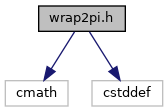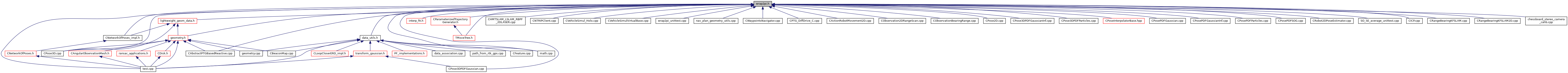MRPT  1.9.9
wrap2pi.h File Reference
`#include <cmath>`
`#include <cstddef>`
Include dependency graph for wrap2pi.h:This graph shows which files directly or indirectly include this file:Go to the source code of this file.

## Namespaces

mrpt::math
This base provides a set of functions for maths stuff.

## Functions

template<class T >
void mrpt::math::wrapTo2PiInPlace (T &a)
Modifies the given angle to translate it into the [0,2pi[ range. More...

template<class T >
mrpt::math::wrapTo2Pi (T a)
Modifies the given angle to translate it into the [0,2pi[ range. More...

template<class T >
mrpt::math::wrapToPi (T a)
Modifies the given angle to translate it into the ]-pi,pi] range. More...

template<class T >
void mrpt::math::wrapToPiInPlace (T &a)
Modifies the given angle to translate it into the ]-pi,pi] range. More...

template<class VECTOR >
void mrpt::math::unwrap2PiSequence (VECTOR &x)
Modify a sequence of angle values such as no consecutive values have a jump larger than PI in absolute value. More...

template<class T >
mrpt::math::angDistance (T from, T to)
Computes the shortest angular increment (or distance) between two planar orientations, such that it is constrained to [-pi,pi] and is correct for any combination of angles (e.g. More...

 Page generated by Doxygen 1.8.14 for MRPT 1.9.9 Git: 7d5e6d718 Fri Aug 24 01:51:28 2018 +0200 at lun nov 2 08:35:50 CET 2020